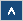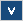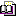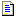Verilog Online HelpTable of ContentsBit-selectBlock StatementsBuilt-in PrimitivesCase StatementContinuous AssignmentsConversion FunctionsCommentsCompiler DirectivesConcatenationsConditional OperatorDelaysDisable StatementDisplay TasksEdge Sensitive PathExpression Bit LengthFile I/O FunctionsFunctionsIdentifiersIf StatementInteger ConstantsIntra-assignment Timing ControlsKeywordsLoop StatementsMemoriesmin:typ:max DelaysModule DeclarationModule InstantiationModule Path DeclarationModule Path PolarityNet Data TypesOperatorsParametersPart-selectPLA Modeling TasksProbabilistic Distribution FunctionsProcedural AssignmentsProcedural Continuous AssignmentsProcedural Timing ControlRange SpecificationReal ConstantsRegister Data TypesSimulation Control TasksSimulation Time FunctionsSpecify BlockState Dependent PathStochastic Analysis TasksStrengthsStringsStructured ProceduresTasksTimescale System TasksTiming Check TasksUDP DeclarationUDP InstantiationUDP State TableValue Change Dump (VCD) FileVectors

# Conditional Operator

## Formal Definition

The conditional operator selects an expression for evaluation depending on the value of condition.

## Simplified Syntax

condition ? expression1 : expression2;

## Description

If the condition is evaluated as false (or zero value) then expression2 is evaluated and used as a result of an entire expression. If condition is evaluated as true (or non-zero value) then expression1 is evaluated. In case condition is evaluated as x or z value, then both expresion1 and expression2 are evaluated, and the result is calculated bit by bit on the basis of the following table:

 0 1 x z 0 0 x x x 1 x 1 x x x x x x x z x x x x

Table 5 Results of bit by bit calculation.

If one of the expressions is of real type then the result of the whole expression should be 0 (zero). If expressions have different lengths, then length of an entire expression will be extended to the length of the longer expression. Trailing 0s will be added to the shorter expression.

The conditional operator can be nested (Example 3) and its behavior is identical with the case statement behavior.

## Examples

Example 1

(a) ? 4'b110x : 4'b1000;

If 'a' has a non-zero value then the result of this expression is 4'b110x. If 'a' is 0, then the result of this expression is 4'b1000. If 'a' is x value then the result is 4'b1x0x (this is due to the fact that the result must be calculated bit by bit on the basis of the Table 1).

Example 2

assign data_out = (enable) ? data_reg : 8'bz;

The above example shows modeling tri-state buffers.

Example 3

reg [4:0] mux;
mux = (addr == 2'b00) ? i0 :
((addr == 2'b01) ? i1 :
((addr == 2'b10) ? i2 :
((addr == 2'b11) ? i3 :
4'bz)));
2'b00: mux = i0;
2'b01: mux = i1;
2'b10: mux = i2;
2'b11: mux = i3;
default: mux = 4'bz;
endcase

Two different methods of modeling a multiplexer.

## Important Notes

• Conditional operator can be used for tri-state buffer modeling.

• Conditional operator can be nested (its behavior is identical with the case statement behavior).